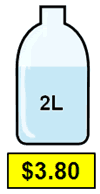# Unit PriceThe "Unit Price" (or "unit cost") tells you the cost per liter, per kilogram, per pound, etc, of what you want to buy. Just divide the cost by the quantity: Example: 2 liters for \$3.80 is \$3.80/2 liters = \$1.90 per liter

## Comparing

Comparing Unit Prices can be a good way of finding which is the "best buy".

Example: What is best

• 2 liters of Milk at \$3.80, or
• 1.5 liters of Milk at \$2.70 ?

In this case the "Unit" is 1 liter, and the Unit Prices are:

• \$3.80 / 2 liters = \$1.90 per liter
• \$2.70 / 1.5 liters = \$1.80 per liter

So the lowest Unit Price (and the best bargain) is 1.5 liters at \$2.70.

Of course it doesn't tell you the quality of what you are buying, but it can help you make a decision.

If something is sold in number of items (for example "10 pencils") then the same method can be used:

Example: What is best

• 10 pencils for \$4.00, or
• 6 pencils for \$2.70 ?

Here is the Unit Cost:

• \$4.00 / 10 = \$0.40 per pencil
• \$2.70 / 6 = \$0.45 per pencil

So the lowest Unit Price (and the best bargain) is 10 pencils for \$4.00

Unit Price Game